# [R Course] Data Visualization with R: Maps

Let’s concentrate on using ggplot2 data for maps and creating maps.

08-16-2019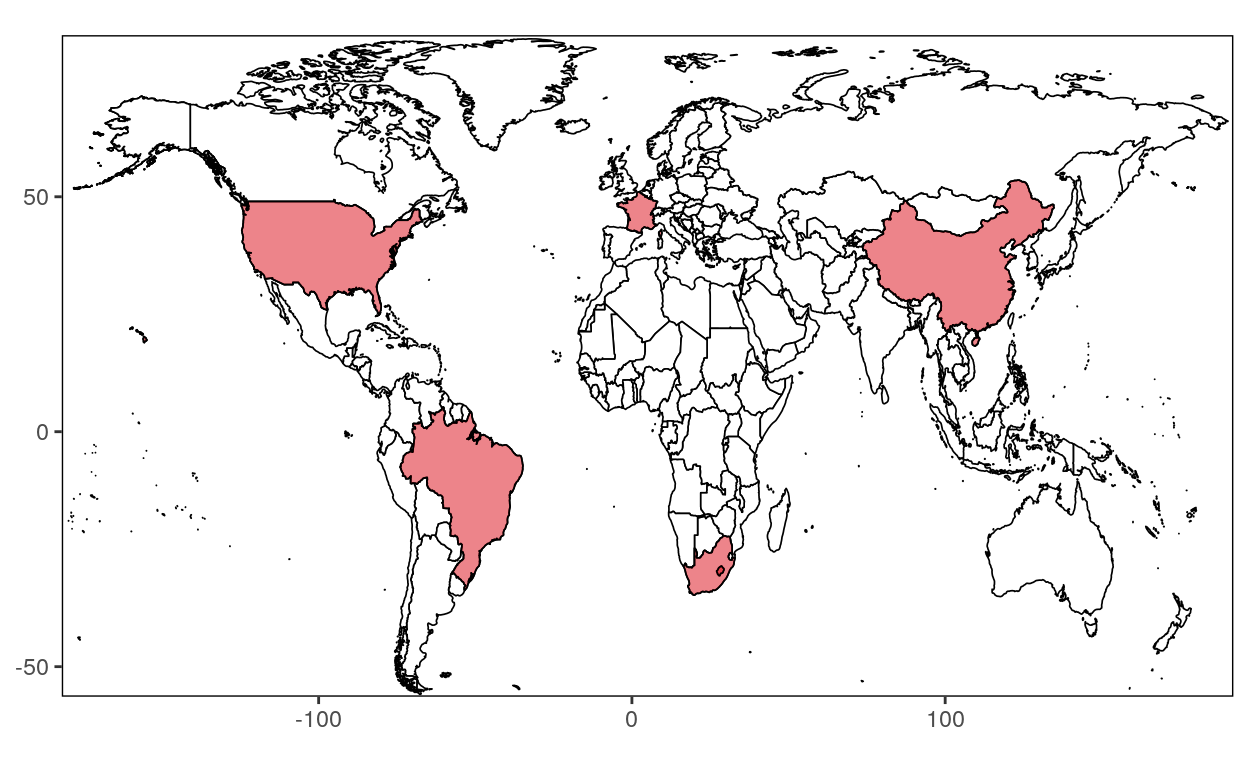There is a multitude ways of getting data to create maps. In the Data Visualization with R course, you’ve seen some of them.

As you know, the `ggplot2` package allows you to produce maps. It also provides data for maps, like longitude and latitude of each countries. It will be easy to turn these data into a data frame suitable for plotting with ggplot2.

## World

``````library(ggplot2)
``````

#### Step 2: Retrieve data

For now, we only use the data of the world to create a map of the world. Later, we’ll see maps for particular region of the world like continents, countries, states or even county!

``````world <- map_data("world")
``````

#### Step 3: Create maps

``````ggplot(data = world, aes(x = long, y = lat, group = group)) +
geom_polygon(fill = "white", color = "black") +
theme_void()
``````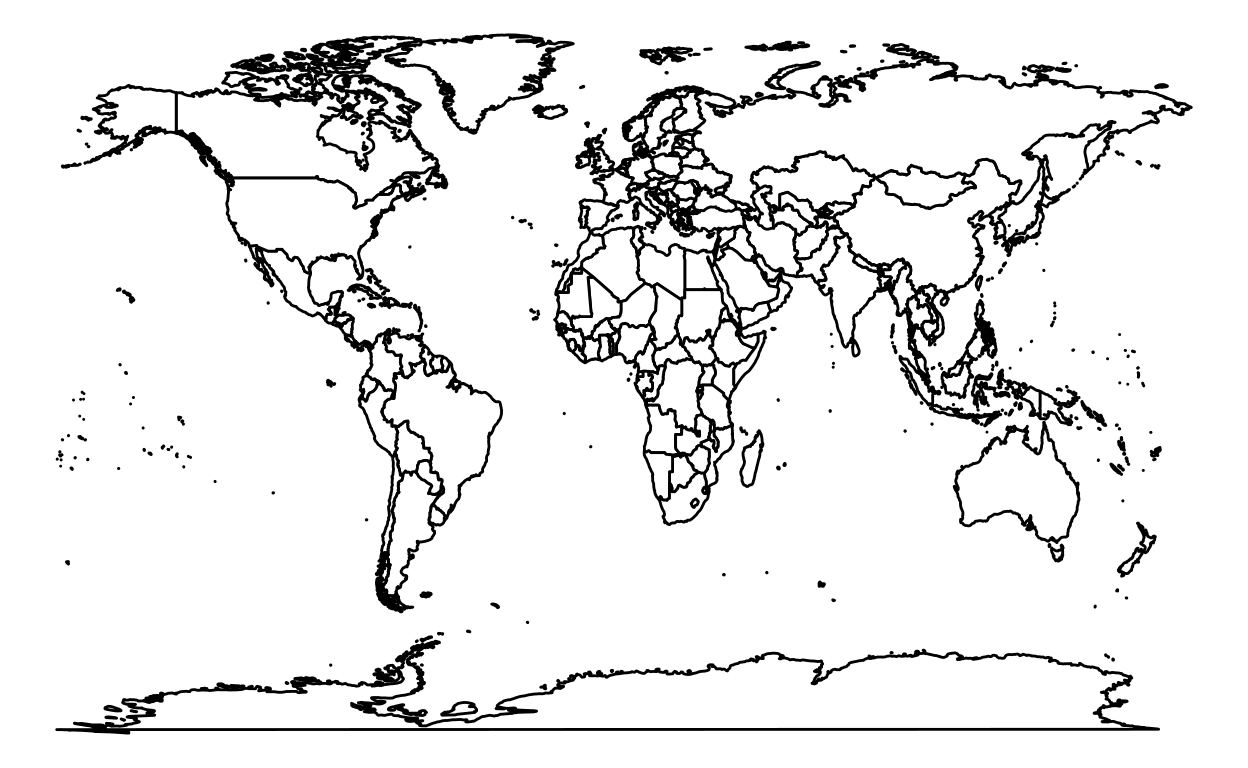## Continents

### Europe

``````library(ggplot2)
``````

#### Step 2: Retrieve data

Getting the world data as in the previous World section.

``````world <- map_data("world")
``````

As you may have guessed, we want to produce a map of Europe. We listed Europe countries to create a map of Europe.

``````europe <- subset(world, region %in% c("Albania", "Andorra", "Armenia", "Austria", "Azerbaijan",
"Belarus", "Belgium", "Bosnia and Herzegovina", "Bulgaria",
"Croatia", "Cyprus", "Czechia","Denmark","Estonia","Finland",
"France","Georgia", "Germany", "Greece","Hungary","Iceland",
"Ireland", "Italy","Kazakhstan", "Kosovo", "Latvia","Liechtenstein",
"Lithuania", "Luxembourg","Malta","Moldova","Monaco","Montenegro",
"Macedonia", "Netherlands","Norway","Poland","Portugal","Romania",
"Russia","San Marino","Serbia","Slovakia","Slovenia","Spain",
"Sweden","Switzerland","Turkey","Ukraine","UK","Vatican"))
``````

#### Step 3: Create maps

``````ggplot(data = europe, aes(x = long, y = lat, group = group)) +
geom_polygon(fill = "white", color = "black") +
theme_void()
``````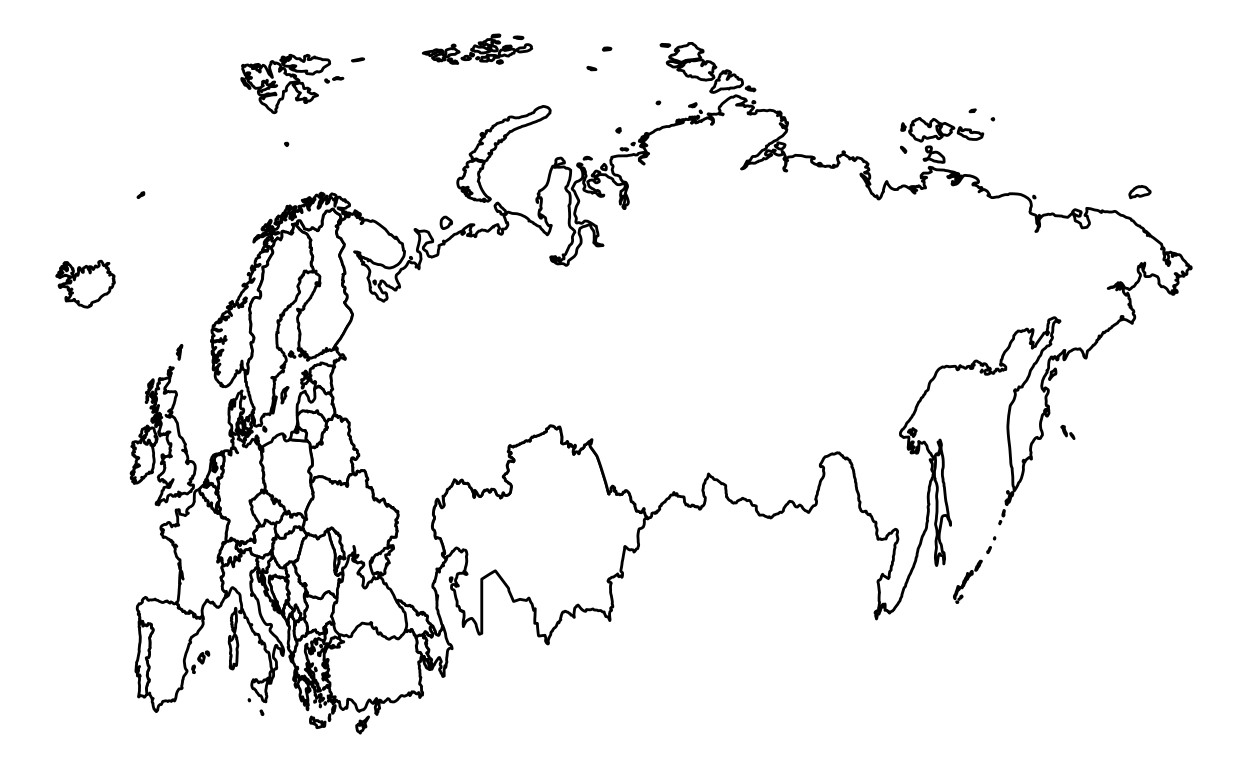The map is too focused on the eastern part. We added some options to refocus the map.

``````ggplot(data = europe, aes(x = long, y = lat, group = group)) +
geom_polygon(fill = "white", color = "black") +
theme_void() +
coord_fixed(ratio=1.6, xlim = c(-50, 80))
``````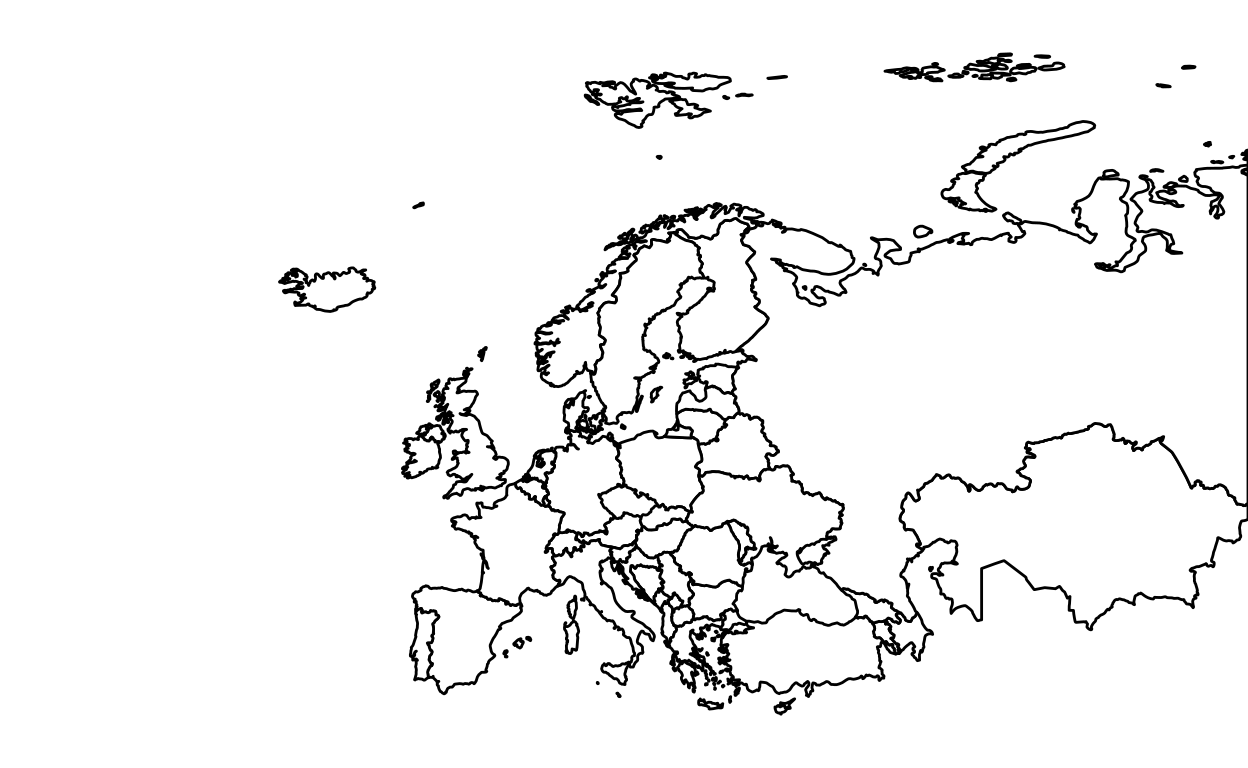We used the `coord_fixed()` function to adjust the plot. The default `ratio` is 1. We used 1.6 as the ration. Honestly, it’s just a “try and error” method. The `xlim` option allows to cut the map horizontally (To cut the map vertically use the ylim option). The number -50 cut the map on the left part of the map. The number 80 cut the map on the right part of the map. Of course, you can cut more or less than 50 on the left side and more or less than 80 for the right side. To see the details of the function.

To keep the entire map without cutting the eastern part like the map above, but with a better focus, here the following code:

``````ggplot(data = europe, aes(x = long, y = lat, group = group)) +
geom_polygon(fill = "white", color = "black") +
theme_void() +
coord_fixed(ratio=1.5, xlim = c(-15,180), ylim = c(35,80))
``````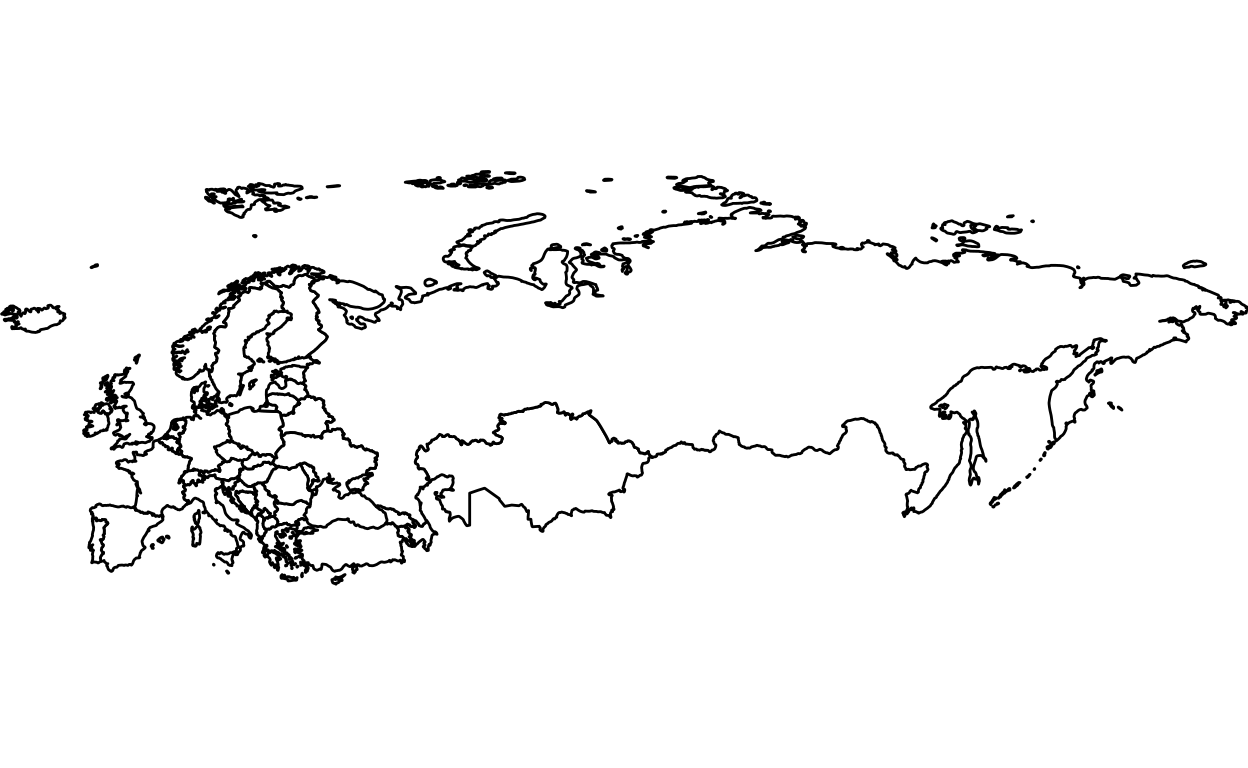### Americas

``````library(ggplot2)
``````

#### Step 2: Retrieve data

``````world <- map_data("world")
americas <- subset(world, region %in% c("USA","Brazil","Mexico", "Colombia", "Argentina", "Canada",
"Honduras", "Paraguay", "Nicaragua","El Salvador", "Costa Rica", "Panama",
"Uruguay",  "Jamaica",  "Trinidad and Tobago", "Guyana", "Suriname", "Belize",
"Antigua and Barbuda", "Saint Kitts and Nevis"))
``````

#### Step 3: Create maps

``````ggplot(data = americas, aes(x = long, y = lat, group = group)) +
geom_polygon(fill = "white", color = "black") +
coord_fixed(ratio=1.1, xlim = c(-180, -35))  +
theme_void()
``````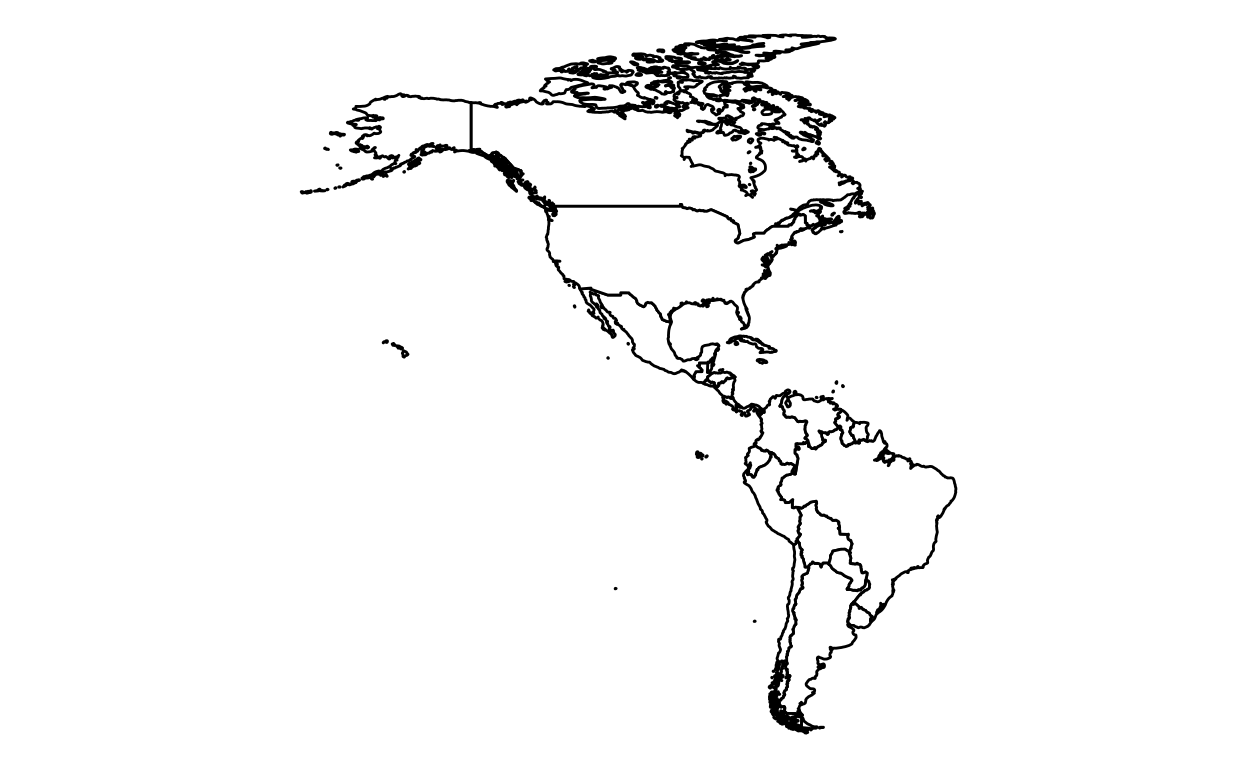### Oceania

``````library(ggplot2)
``````

#### Step 2: Retrieve data

``````world <- map_data("world")
oceania <- subset(world, region %in% c("Australia", "Fiji", "Kiribati", "Marshall Islands", "Micronesia",
"Nauru", "New Zealand", "Palau"," Papua New Guinea", "Samoa",
"Solomon Islands", "Tonga", "Tuvalu", "Vanuatu"))
``````

#### Step 3: Create maps

``````ggplot(data = oceania, aes(x = long, y = lat, group = group)) +
geom_polygon(fill = "white", color = "black") +
coord_fixed(xlim = c(110,180)) +
theme_void()
``````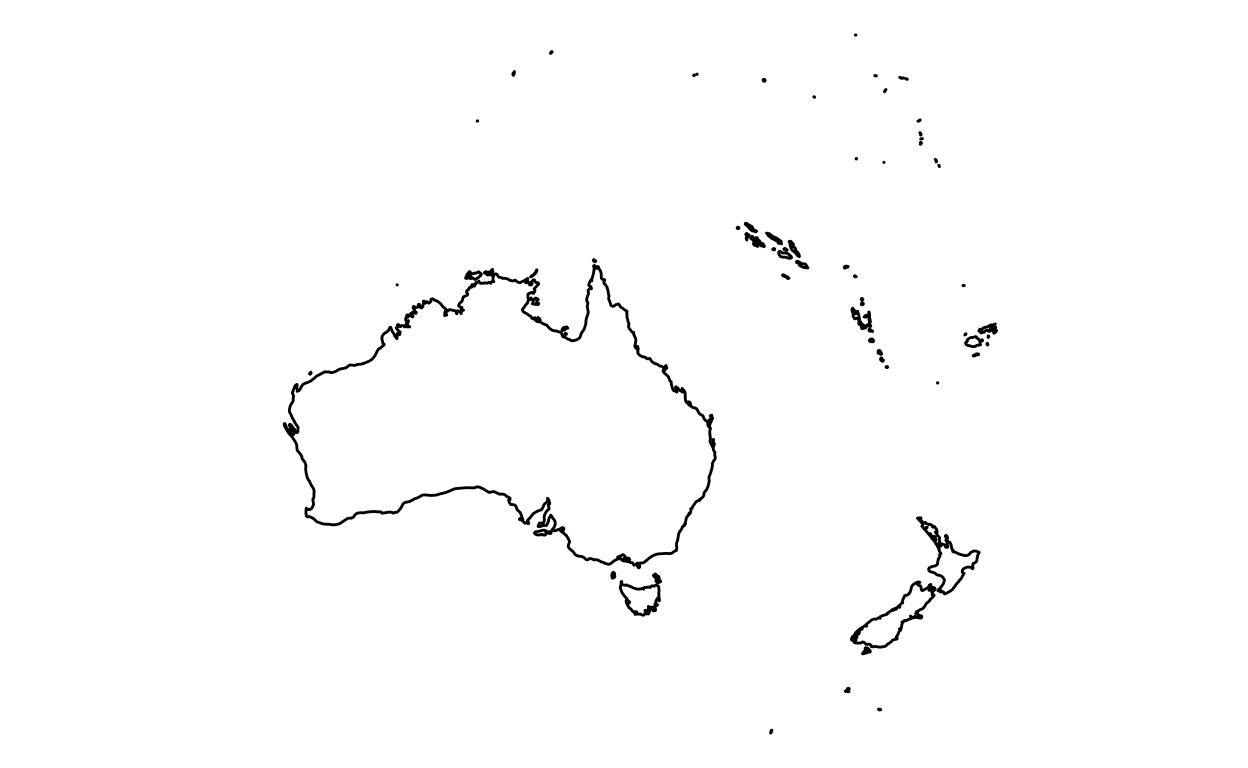### Asia

``````library(ggplot2)
``````

#### Step 2: Retrieve data

``````world <- map_data("world")
asia <- subset(world, region %in% c("Afghanistan", "Armenia", "Azerbaijan", "Bahrain", "Bangladesh", "Bhutan",
"Brunei", "Cambodia", "China", "Cyprus", "Georgia", "India", "Indonesia",
"Iran", "Iraq", "Israel", "Japan", "Jordan", "Kazakhstan", "Kuwait",
"Kyrgyzstan", "Laos", "Lebanon", "Malaysia", "Maldives", "Mongolia", "Myanmar",
"Nepal", "North Korea", "Oman", "Pakistan", "Palestine", "Philippines", "Qatar",
"Russia", "Saudi Arabia", "Singapore", "South Korea", "Sri Lanka", "Syria",
"Taiwan", "Tajikistan", "Thailand", "Timor-Leste", "Turkey", "Turkmenistan",
"United Arab Emirates", "Uzbekistan", "Vietnam", "Yemen"))
``````

#### Step 3: Create maps

``````ggplot(data = asia, aes(x = long, y = lat, group = group)) +
geom_polygon(fill = "white", color = "black") +
coord_fixed(1.2) +
theme_void()
``````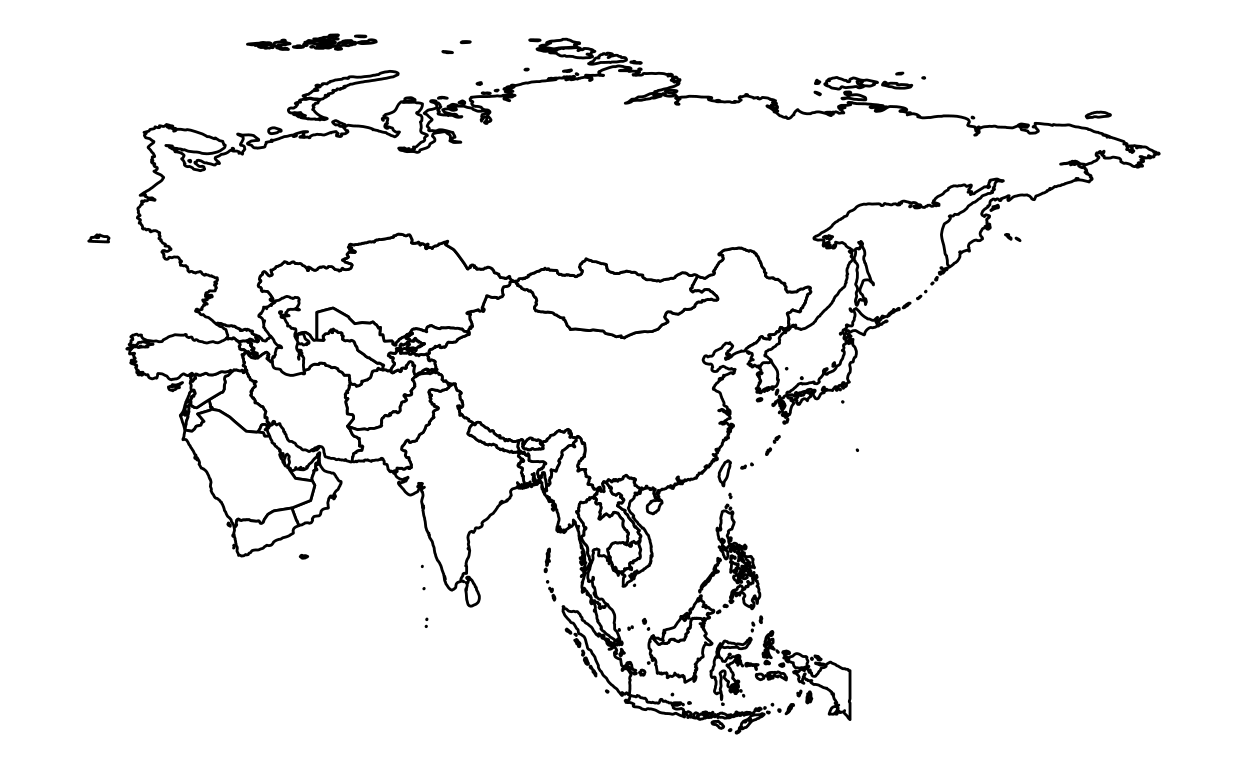### Africa

``````library(ggplot2)
``````

#### Step 2: Retrieve data

``````world <- map_data("world")
africa <- subset(world, region %in% c("Algeria","Angola","Benin","Botswana","Burkina Faso","Burundi",
"Democratic Republic of the Congo","Republic of Congo","Ivory Coast",
"Djibouti","Egypt","Equatorial Guinea","Eritrea","Swaziland","Ethiopia",
"Gabon","Gambia","Ghana","Guinea","Guinea-Bissau","Kenya","Lesotho","Liberia",
"Mozambique","Namibia","Niger","Nigeria","Rwanda","Sao Tome and Principe",
"Senegal","Seychelles","Sierra Leone","Somalia","South Africa","South Sudan",
"Sudan","Tanzania","Togo","Tunisia","Uganda","Zambia","Zimbabwe"))
``````

#### Step 3: Create maps

``````ggplot(data = africa, aes(x = long, y = lat, group = group)) +
geom_polygon(fill = "white", color = "black") +
coord_fixed() +
theme_void()
``````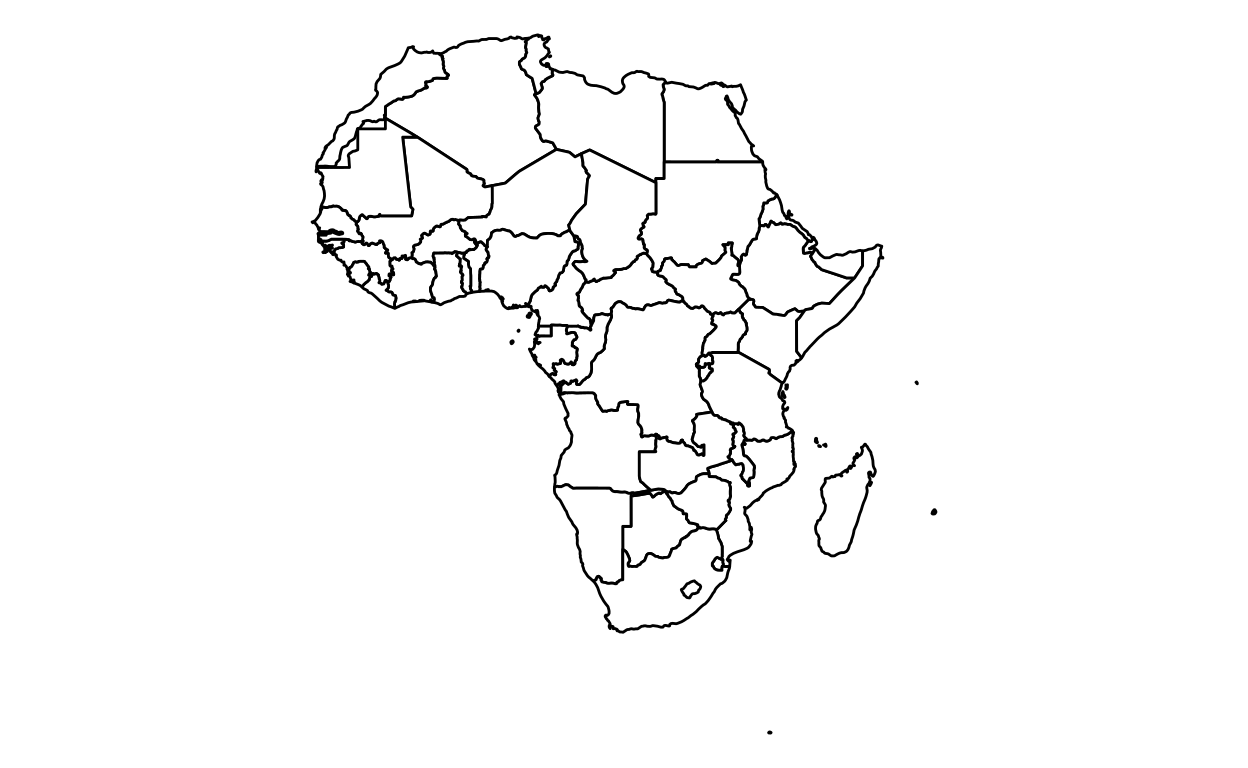To color a country (in this case Nigeria):

``````nigeria <- subset(world, region %in% c("Nigeria"))
``````
``````ggplot(data = africa, aes(x = long, y = lat, group = group)) +
geom_polygon(data = africa, fill = "white", color = "black") +
geom_polygon(data = nigeria, fill = "gray", color = "black") +
coord_fixed() +
theme_void()
``````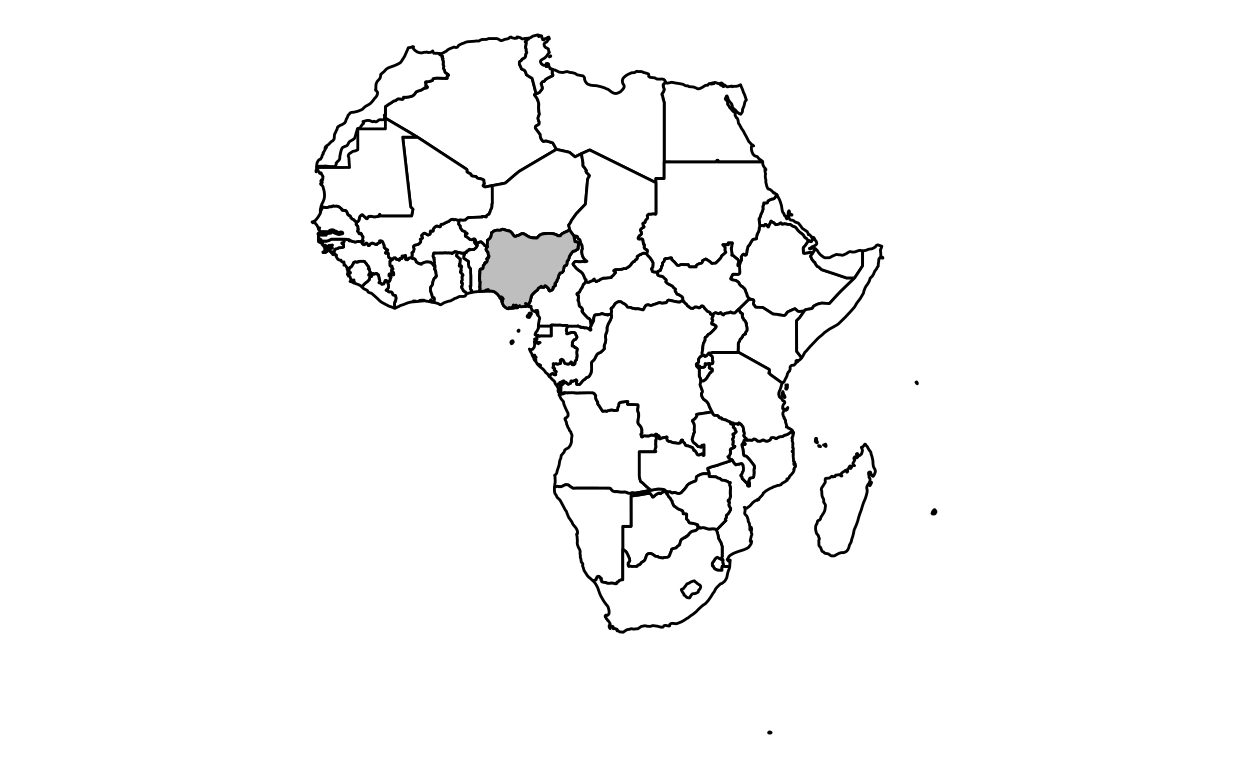## Countries

### Nigeria

To color a specific country in a map, here how to do it:

``````library(ggplot2)
``````

#### Step 2: Retrieve data

``````nigeria <- map_data("world", region = "Nigeria")
``````

#### Step 3: Create maps

To only keep the map of Nigeria:

``````ggplot(data = nigeria, aes(x = long, y = lat, group = group)) +
geom_polygon(fill = "white", color = "black") +
coord_fixed() +
theme_void()
``````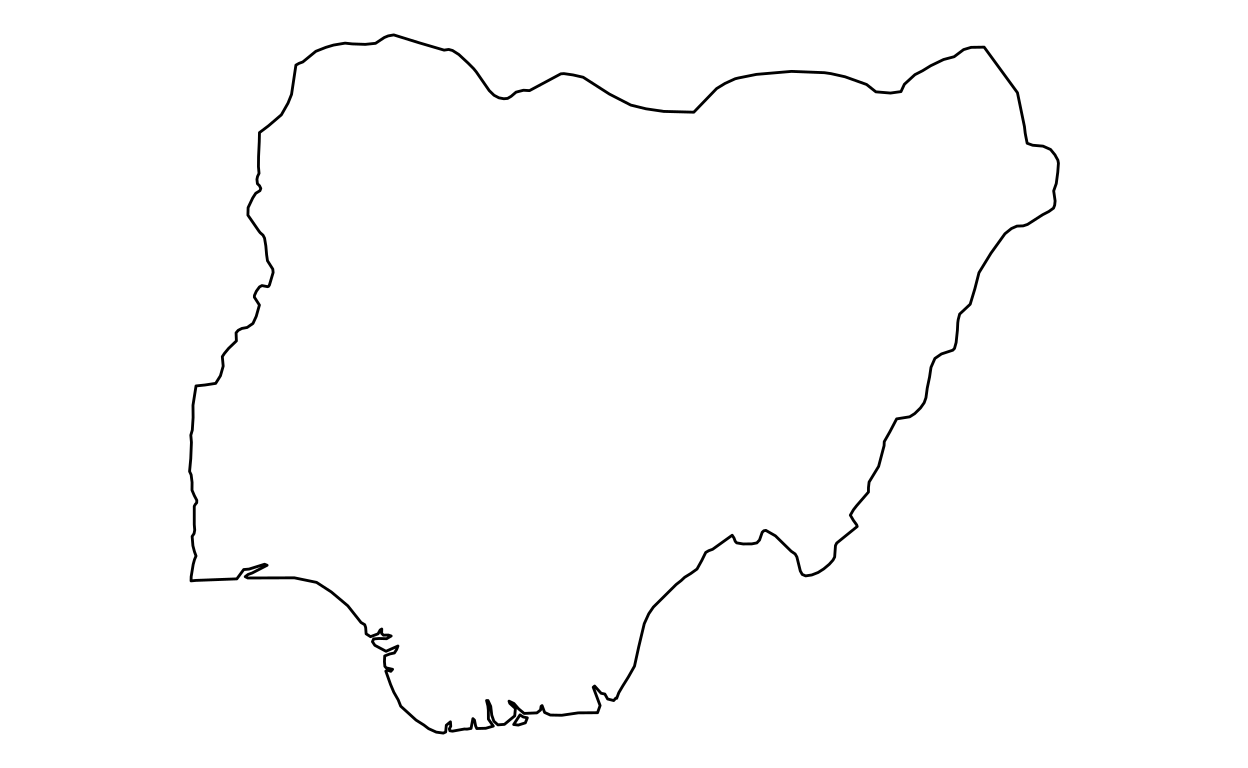``````library(ggplot2)
``````

#### Step 2: Retrieve data

``````canada <- map_data("world", region = "Canada")
``````

#### Step 3: Create maps

``````ggplot(data=canada, aes(x = long, y = lat, group = group)) +
geom_polygon(fill = "white", color="black")+
coord_fixed(1.8) +
theme_void()
``````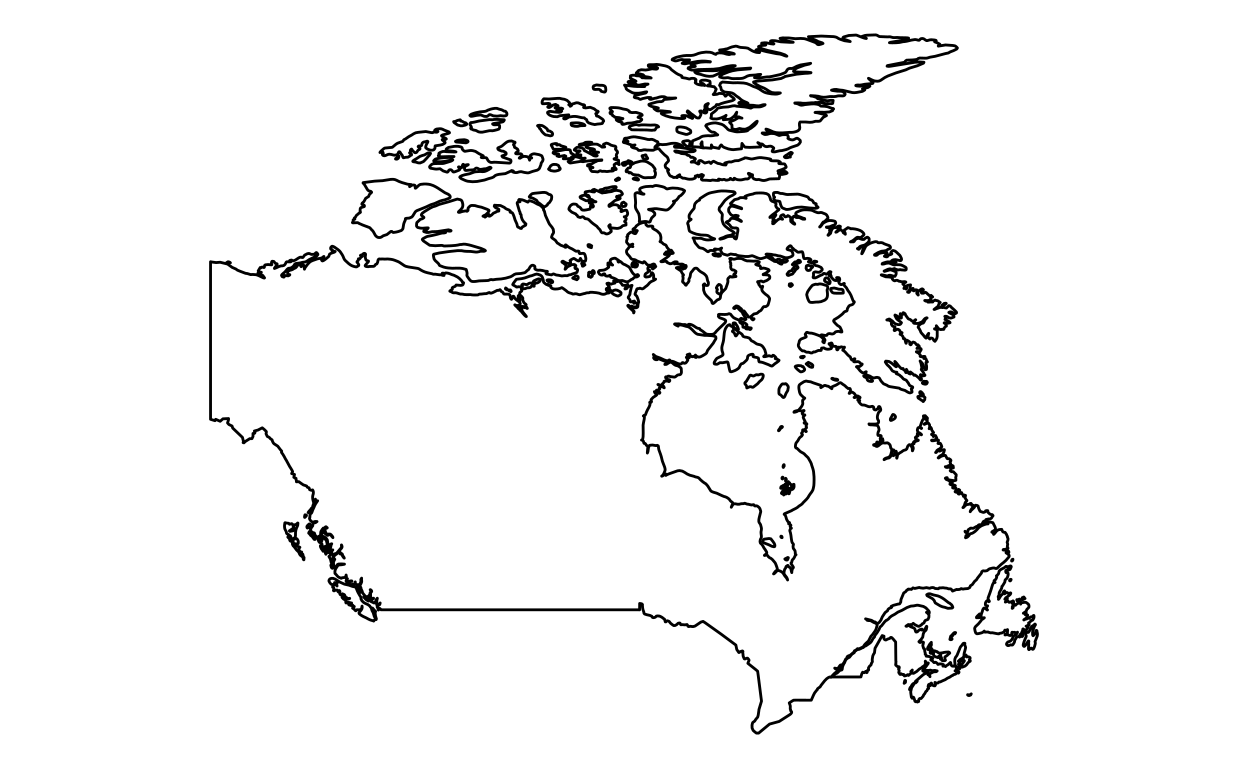### China

``````library(ggplot2)
``````

#### Step 2: Retrieve data

``````china <- map_data("world", region = "China")
``````

#### Step 3: Create maps

``````ggplot(china, aes(x = long, y = lat, group = group)) +
geom_polygon(fill = "white", color="black")+
coord_fixed(1.3) +
theme_void()
``````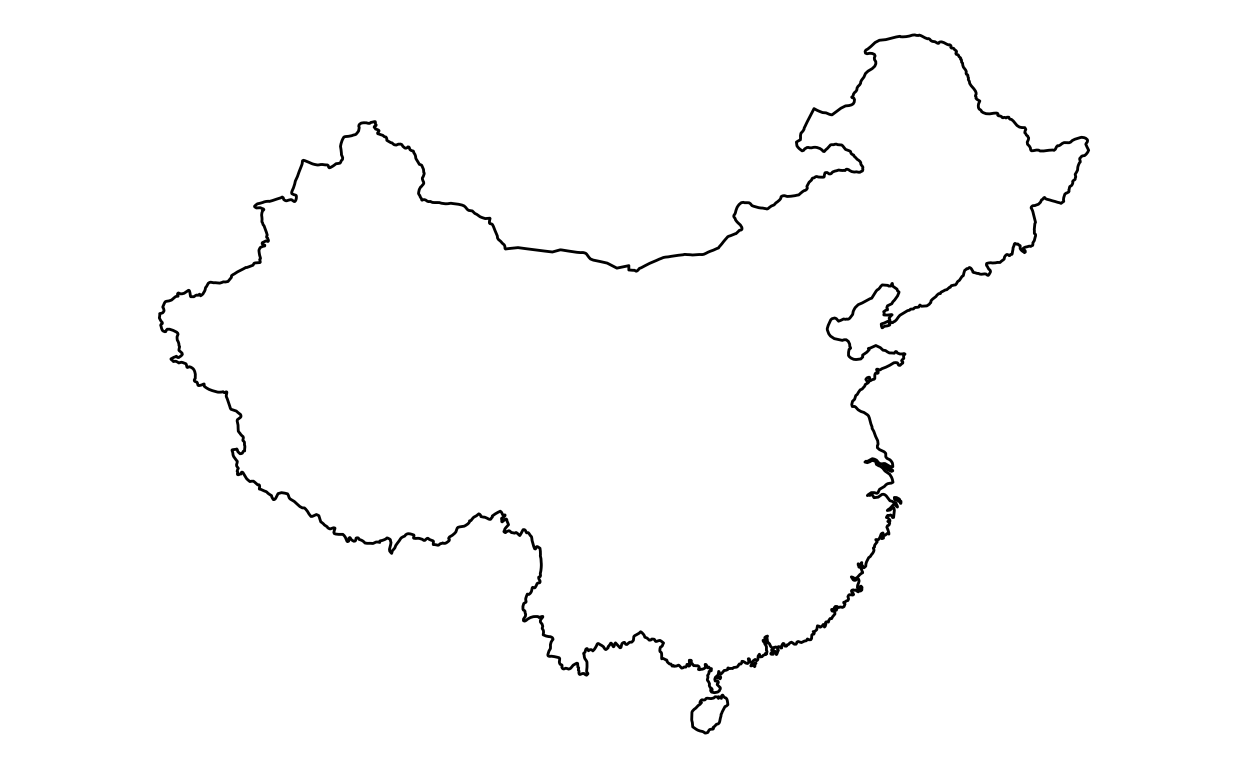### United States

``````library(ggplot2)
library(cowplot)
``````

#### Step 2: Retrieve data

``````usa <- map_data("world", region = "USA")
``````

#### Step 3: Create maps

``````ggplot(data=usa, aes(x = long, y = lat, group = group)) +
geom_polygon(fill = "white", color = "black")+
coord_fixed(1.8, xlim = c(-180,0)) +
theme_void()
``````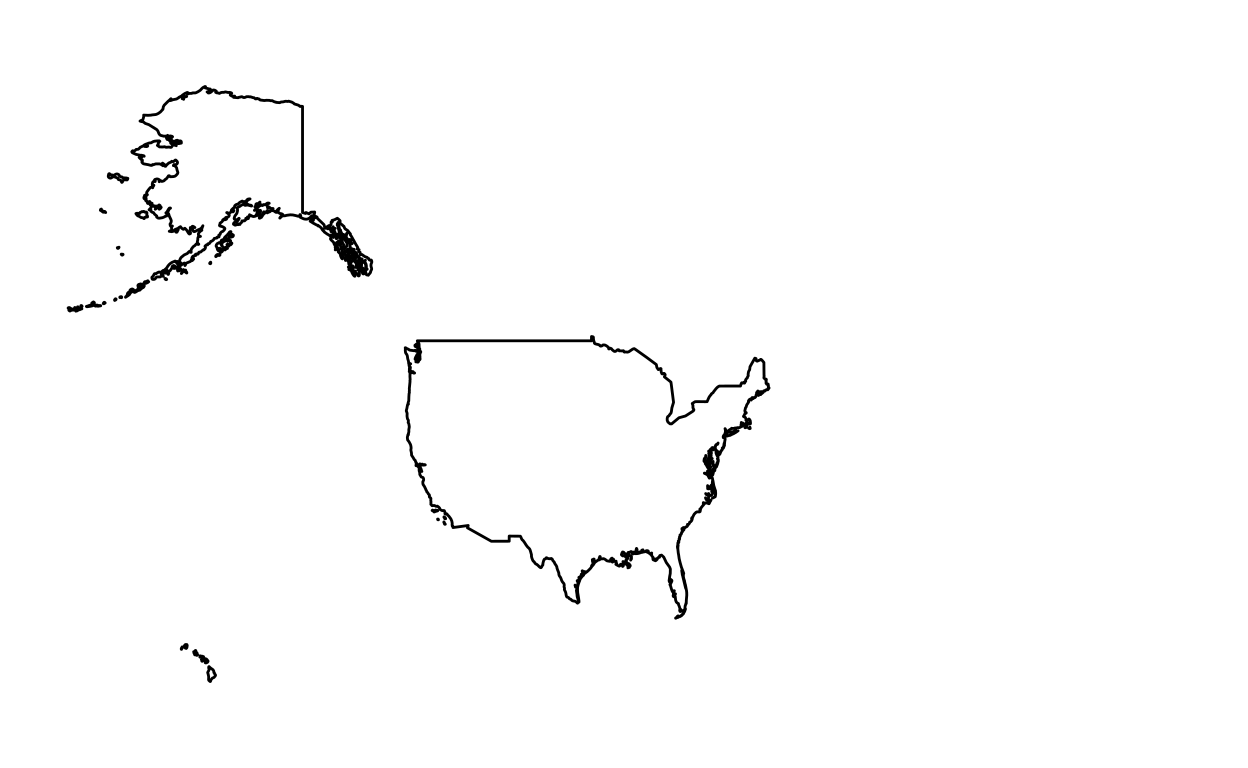If you don’t like the appearance of the map, here an alternative.

1. We only keep the mainland:
``````(mainland <- ggplot(data=usa, aes(x = long, y = lat, group = group)) +
geom_polygon(fill = "white", color = "black")+
coord_fixed(1.5, xlim = c(-125,-68), ylim = c(50,22)) +
theme_void())
``````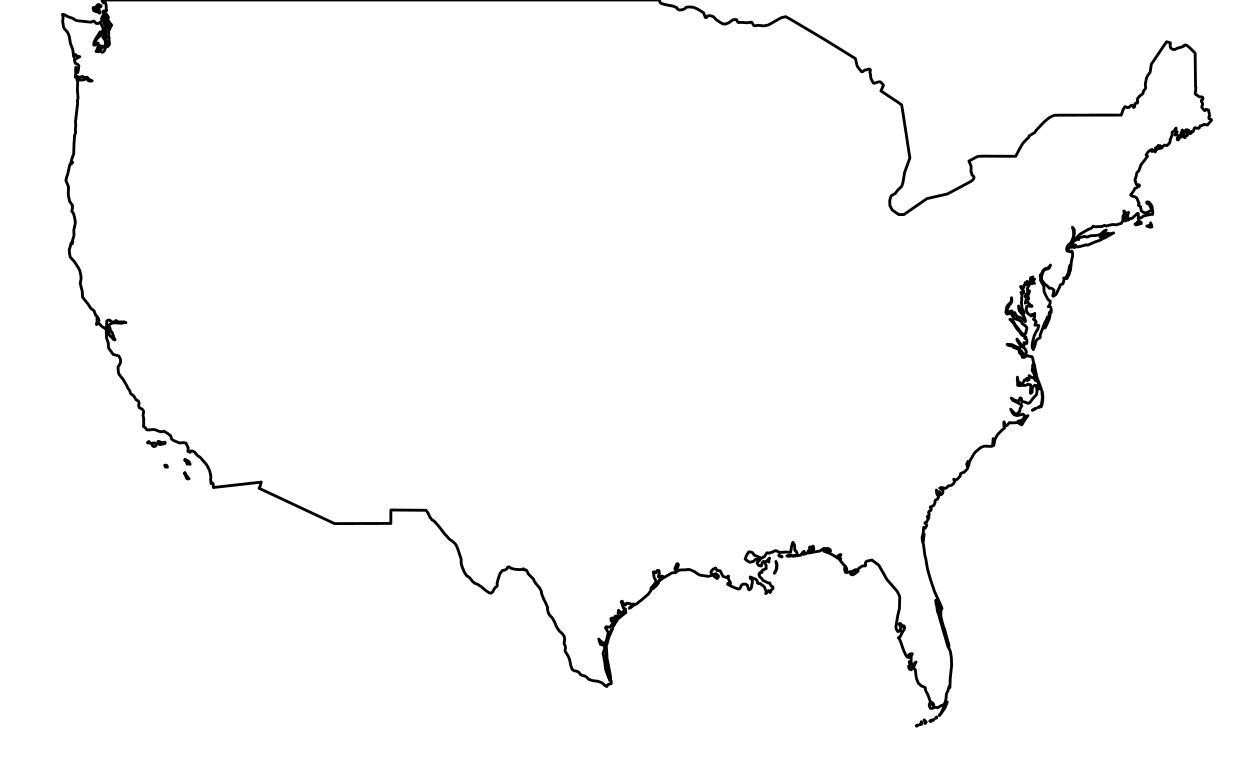``````(alaska <- ggplot(data = usa, aes(x = long, y = lat, group = group)) +
geom_polygon(fill = "white", color = "black") +
coord_fixed(1.6, xlim = c(-178,-132), ylim = c(52,71)) +
theme_void())
``````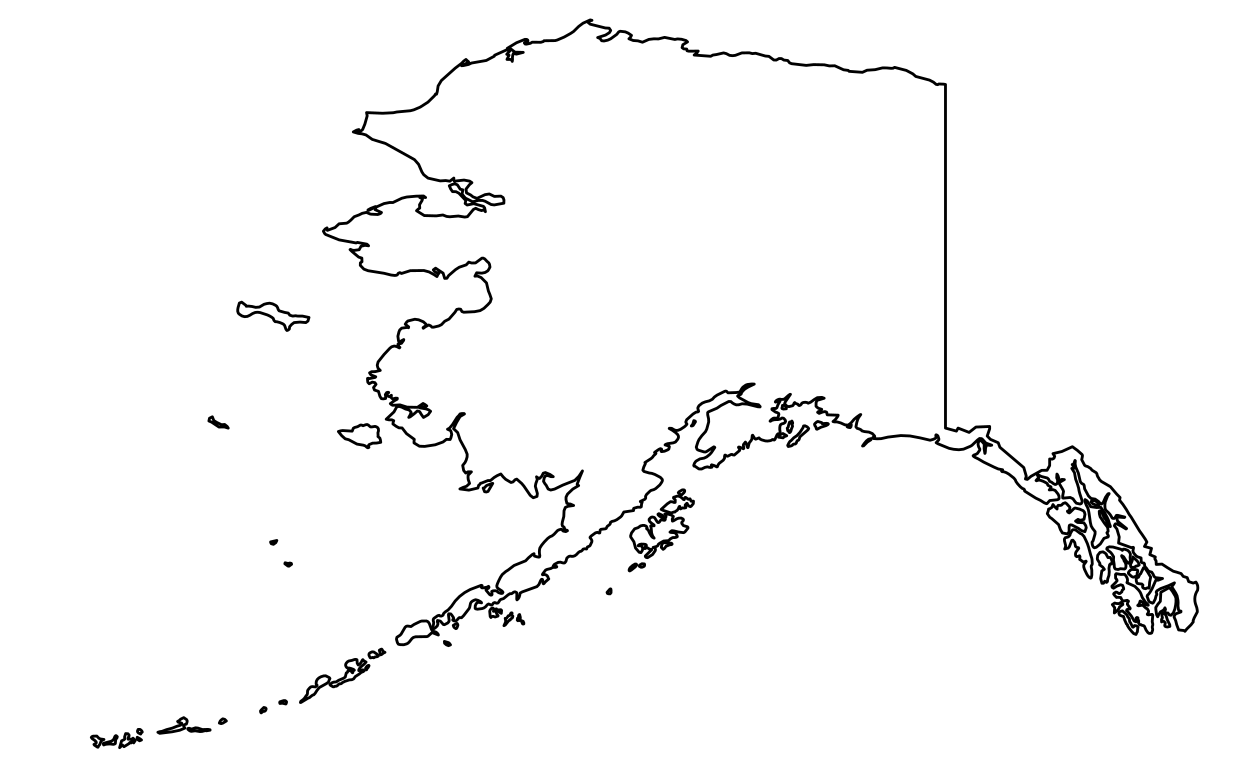1. We keep Hawaii:
``````(hawaii <- ggplot(data=usa, aes(x = long, y = lat, group = group)) +
geom_polygon(fill = "white", color = "black") +
coord_fixed(1.5, xlim = c(-162,-153), ylim = c(23,18)) +
theme_void())
``````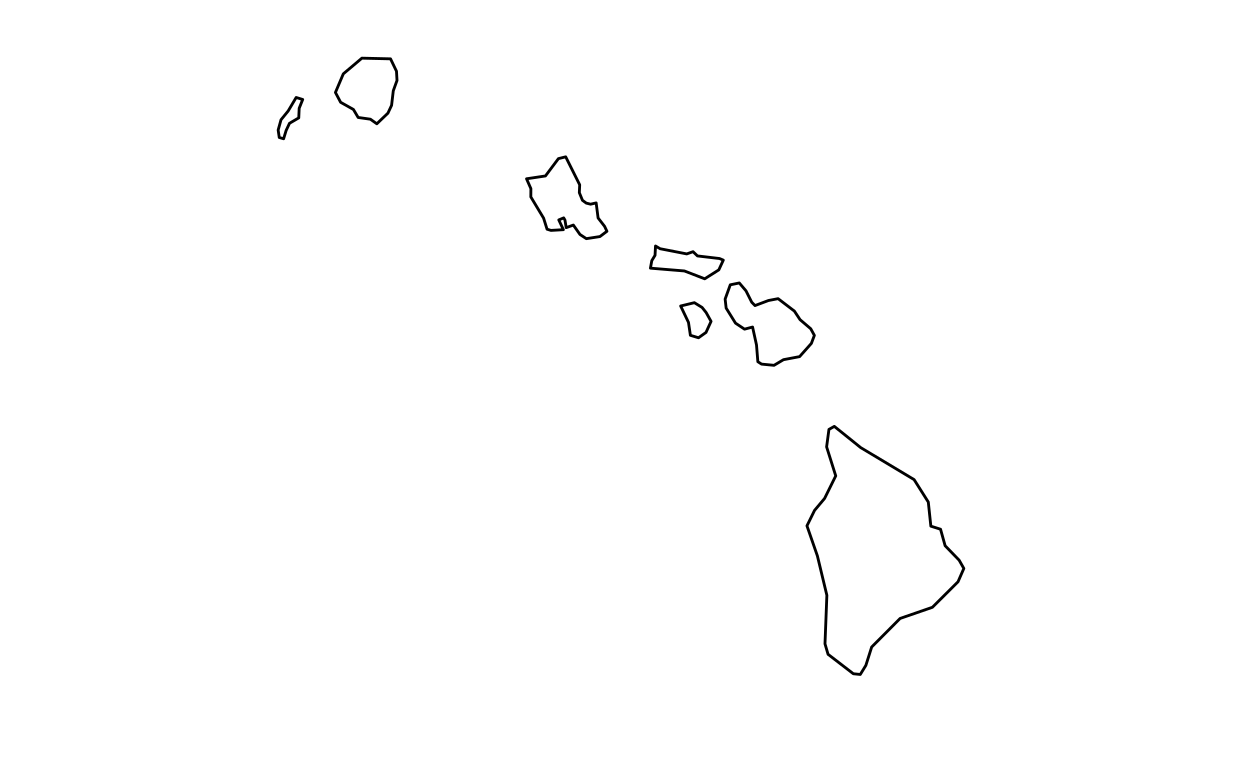1. We put it together:
``````ratioAlaska <- (25 - 2) / (16 - (-24))
ratioHawaii  <- (23 - 18) / (-154 - (-161))

ggdraw(mainland) +
draw_plot(alaska, width = 0.26, height = 0.26 * 10/6 * ratioAlaska, x = 0.1, y = 0.01) +
draw_plot(hawaii, width = 0.15, height = 0.15 * 10/6 * ratioHawaii, x = 0.3, y = 0.01)
``````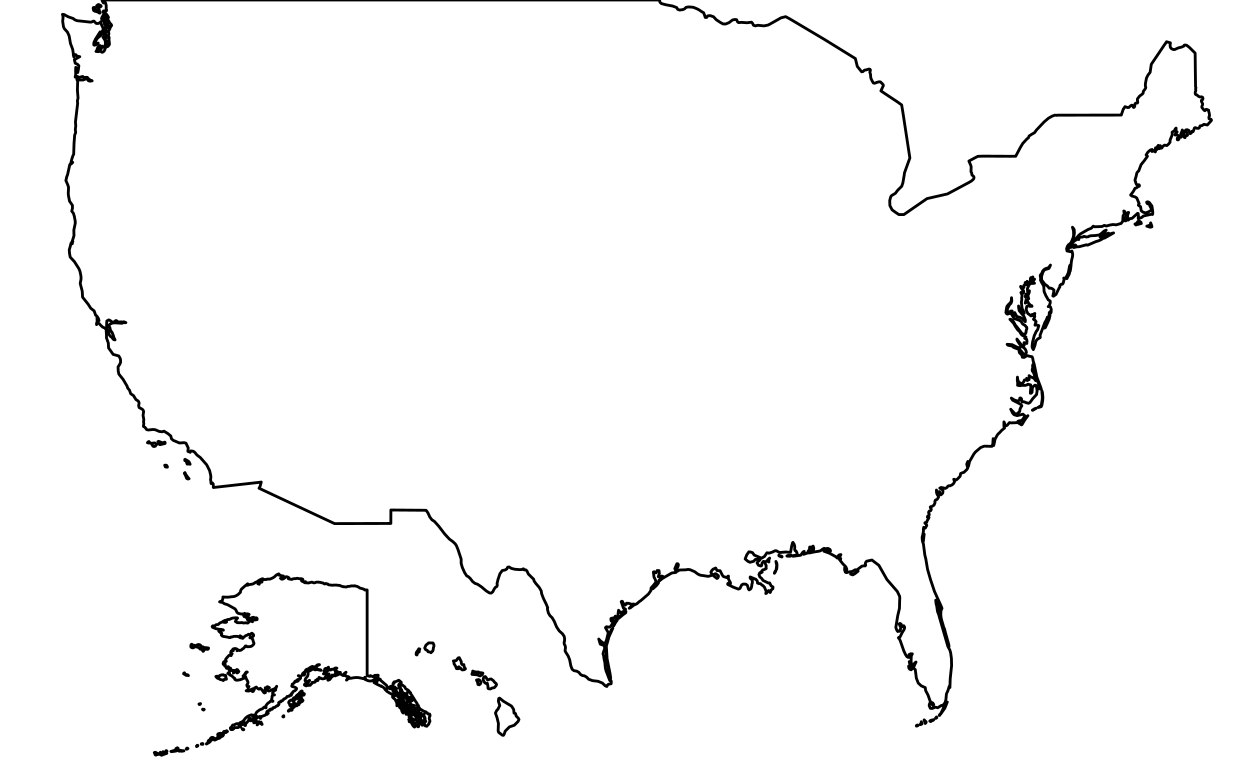## States

### All US states

``````library(ggplot2)
``````

#### Step 2: Retrieve data

``````usa <- map_data("world", region = "USA")

usa_mainland <- subset(usa, subregion != "Alaska")
usa_mainland <- subset(usa_mainland, subregion != "Hawaii")

usa_states <- map_data("state")
``````

#### Step 3: Create maps

``````ggplot(data = usa_mainland, aes(x = long, y = lat, group = group)) +
geom_polygon(data = usa_states, fill = "white", color = "black") +
coord_fixed(1.4) +
theme_void()
``````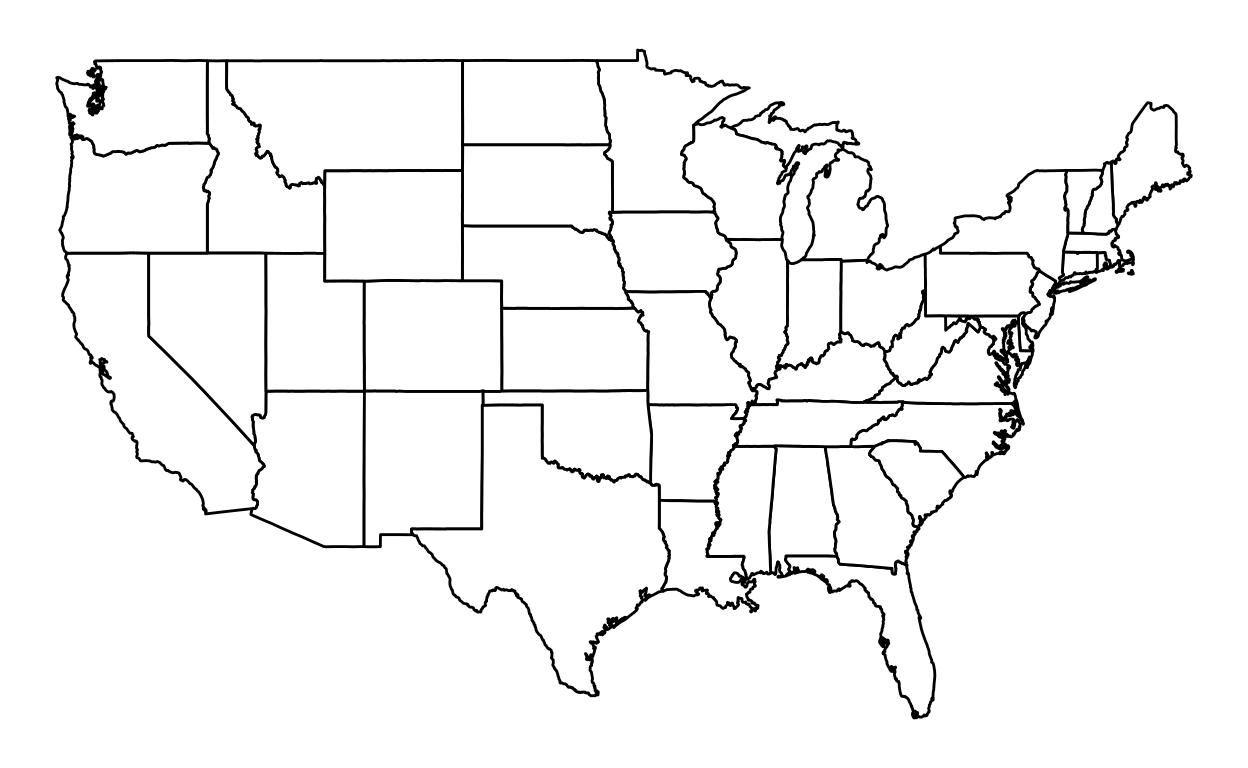To color a state:

``````nevada <- subset(usa_states, region == "nevada")
``````
``````ggplot(data = usa_states, aes(x = long, y = lat, group = group)) +
geom_polygon(data = usa_states, fill = "white", color = "black") +
geom_polygon(data = nevada, fill = "gray", color = "black") +
coord_fixed(1.4) +
theme_void()
``````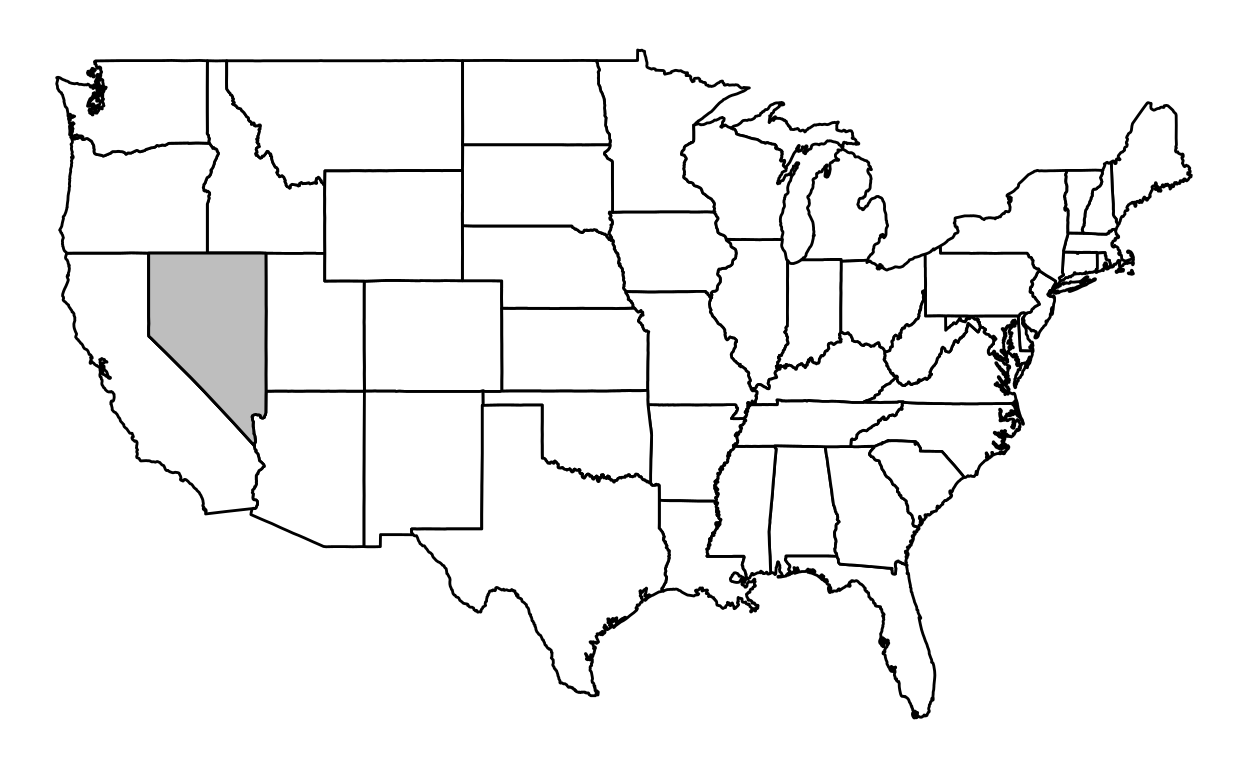``````library(ggplot2)
``````

#### Step 2: Retrieve data

``````nevada <- subset(usa_states, region == "nevada")
``````

#### Step 3: Create maps

``````ggplot(data = nevada, aes(x = long, y = lat, group = group)) +
geom_polygon(fill = "white", color = "black") +
coord_fixed(1.4) +
theme_void()
``````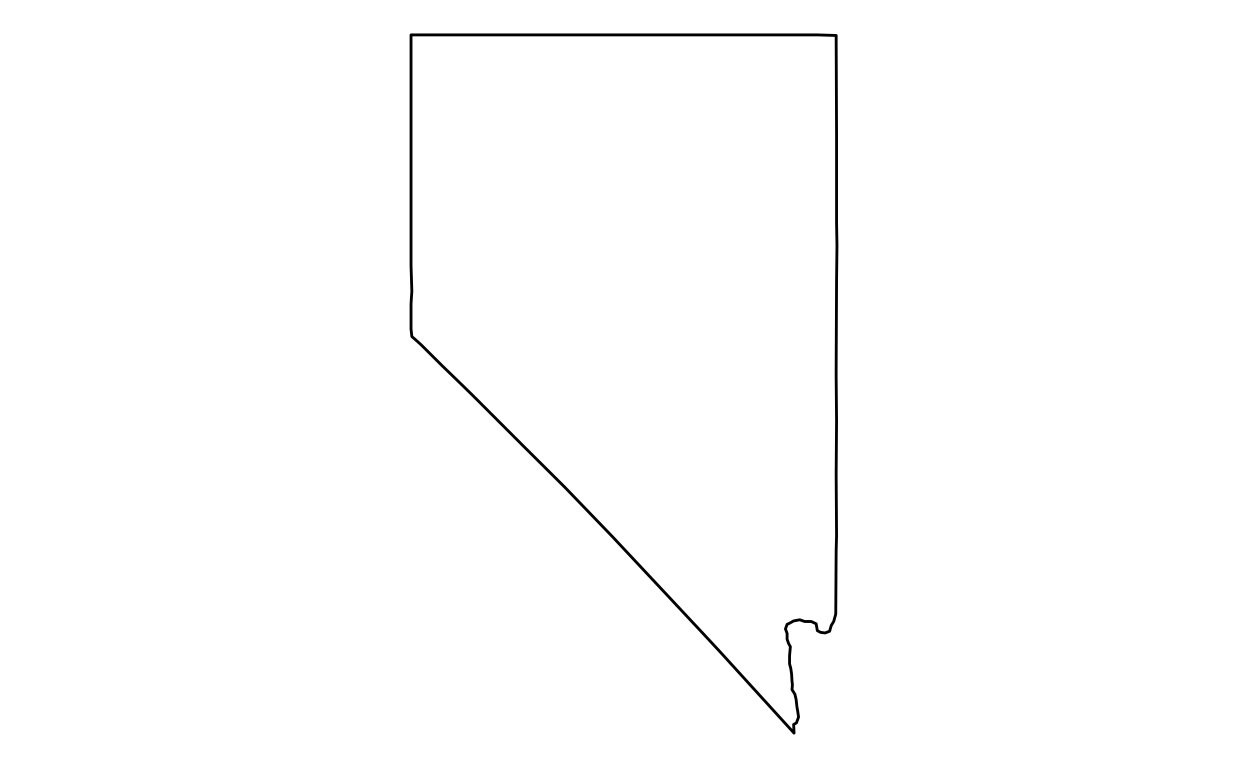### Texas

``````library(ggplot2)
``````

#### Step 2: Retrieve data

``````texas <- subset(usa_states, region == "texas")
``````

#### Step 3: Create maps

To only keep a state (in this case Texas):

``````ggplot(data = texas, aes(x = long, y = lat, group = group)) +
geom_polygon(fill = "white", color = "black") +
coord_fixed(1.4) +
theme_void()
``````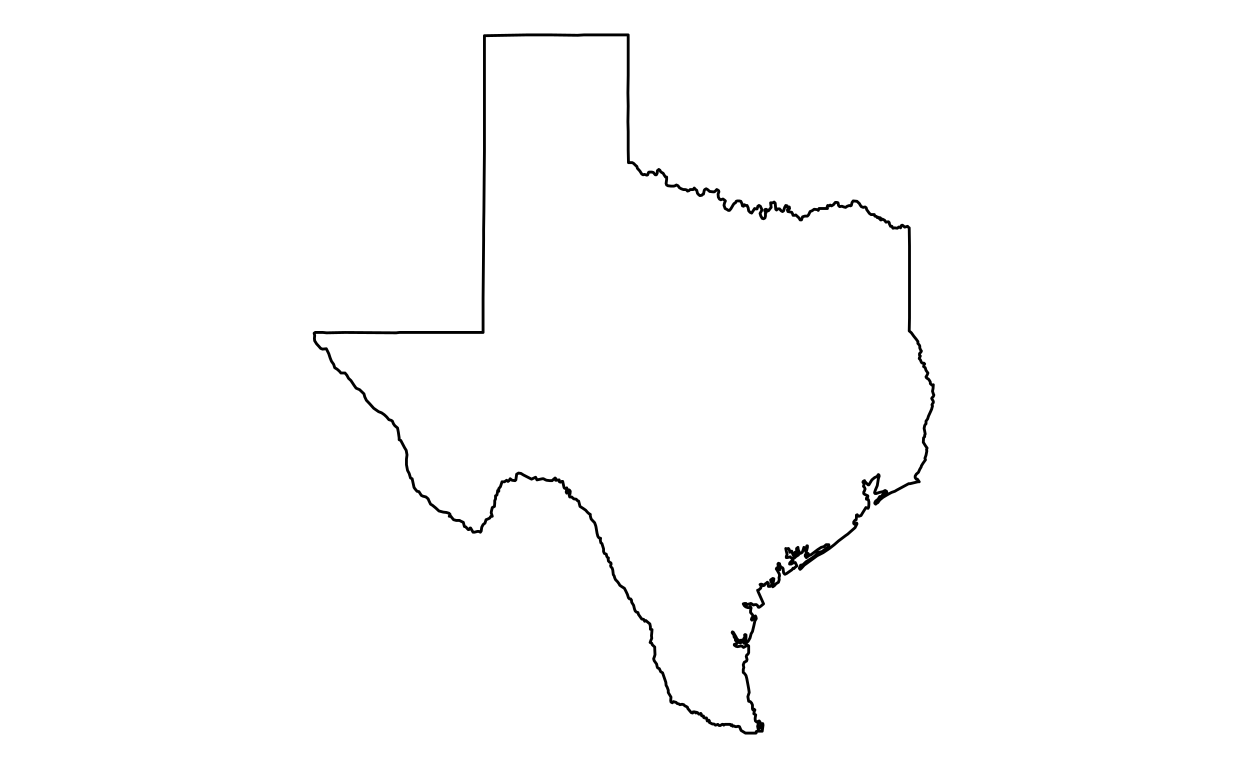### California

``````library(ggplot2)
``````

#### Step 2: Retrieve data

``````california <- subset(usa_states, region == "california")
``````

#### Step 3: Create maps

``````ggplot(data = california, aes(x = long, y = lat, group = group)) +
geom_polygon(fill = "white", color = "black") +
coord_fixed(1.4) +
theme_void()
``````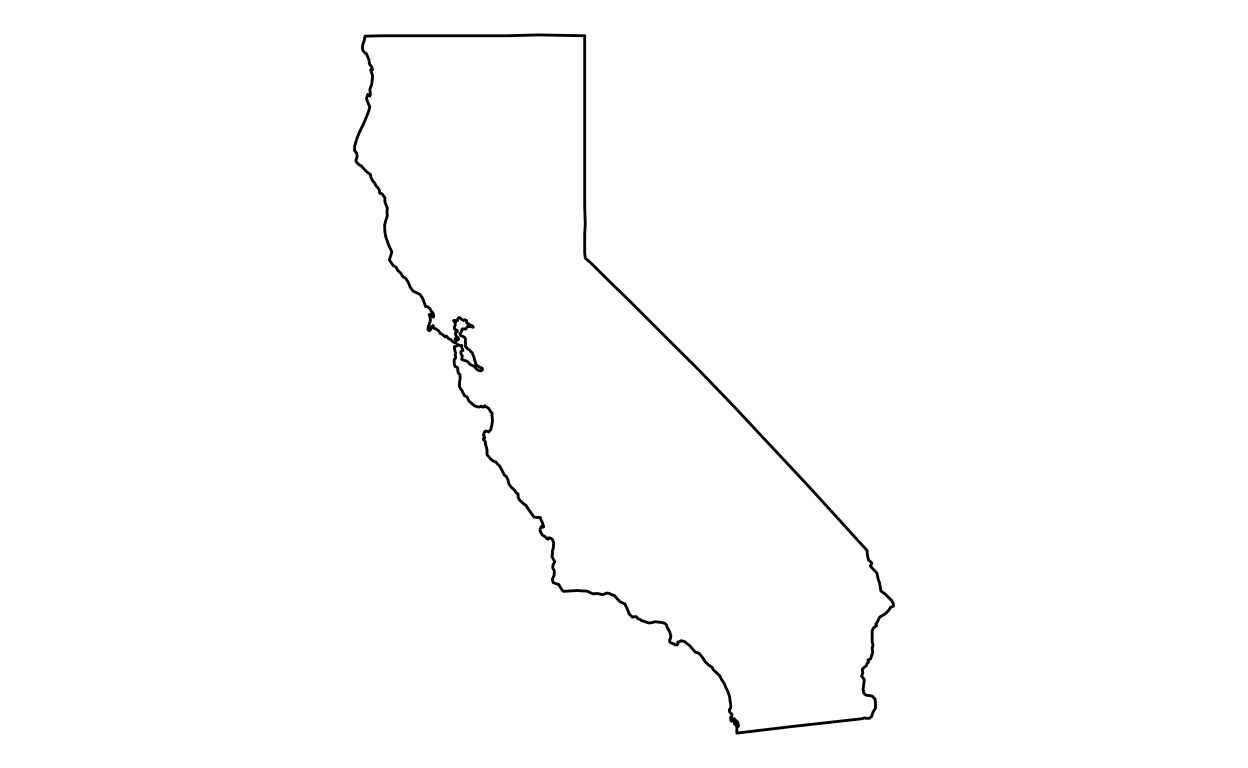## County

### Los Angeles

``````library(ggplot2)
``````

#### Step 2: Retrieve data

``````usa_states <- map_data("state")
california <- subset(usa_states, region %in% c("california"))

usa_counties <- map_data("county")
california_counties <- subset(usa_counties, region == "california")

losAngeles <- subset(california_counties, subregion == "los angeles")
``````

#### Step 3: Create maps

``````ggplot(data = california, aes(x = long, y = lat, group = group)) +
geom_polygon(data = california_counties, fill = "white", color = "black") +
geom_polygon(data = losAngeles, fill = "gray", color = "black") +
coord_fixed(1.4) +
theme_void()
``````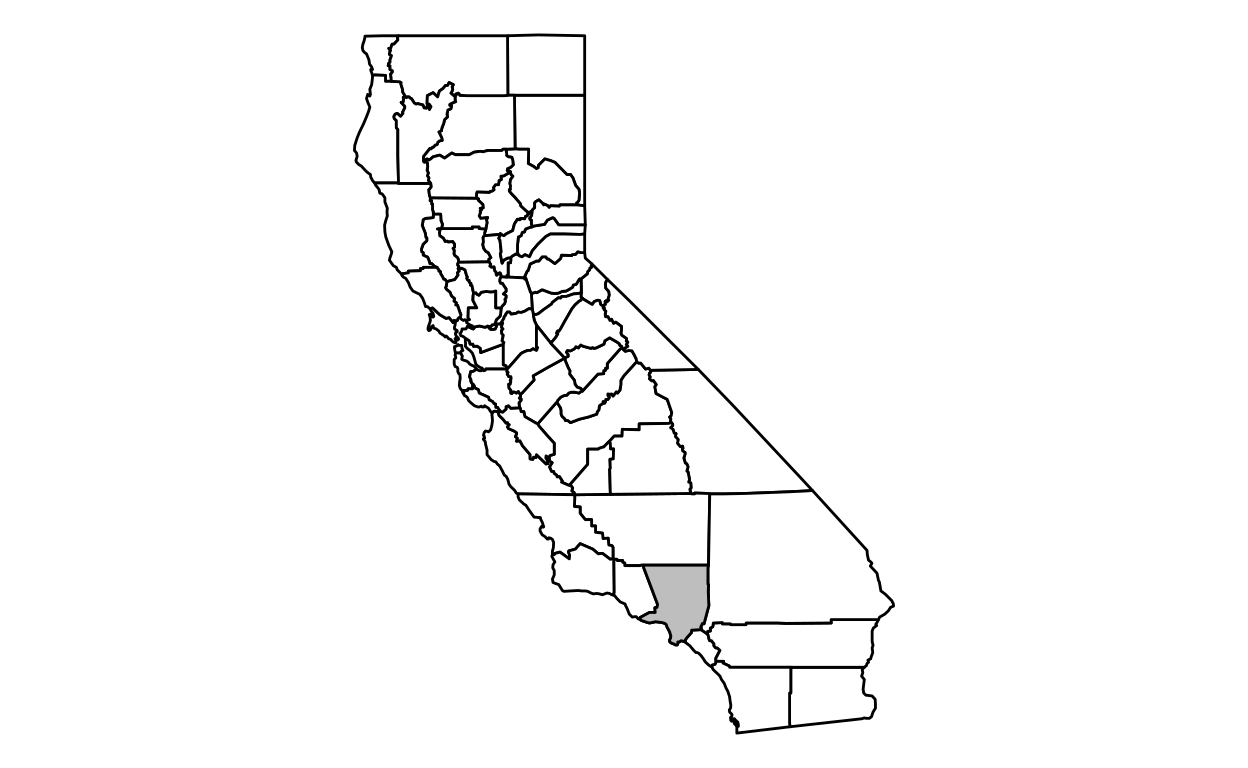To only keep the county map:

``````ggplot(data = losAngeles, aes(x = long, y = lat, group = group)) +
geom_polygon(fill = "white", color = "black") +
coord_fixed(1.4) +
theme_void()
``````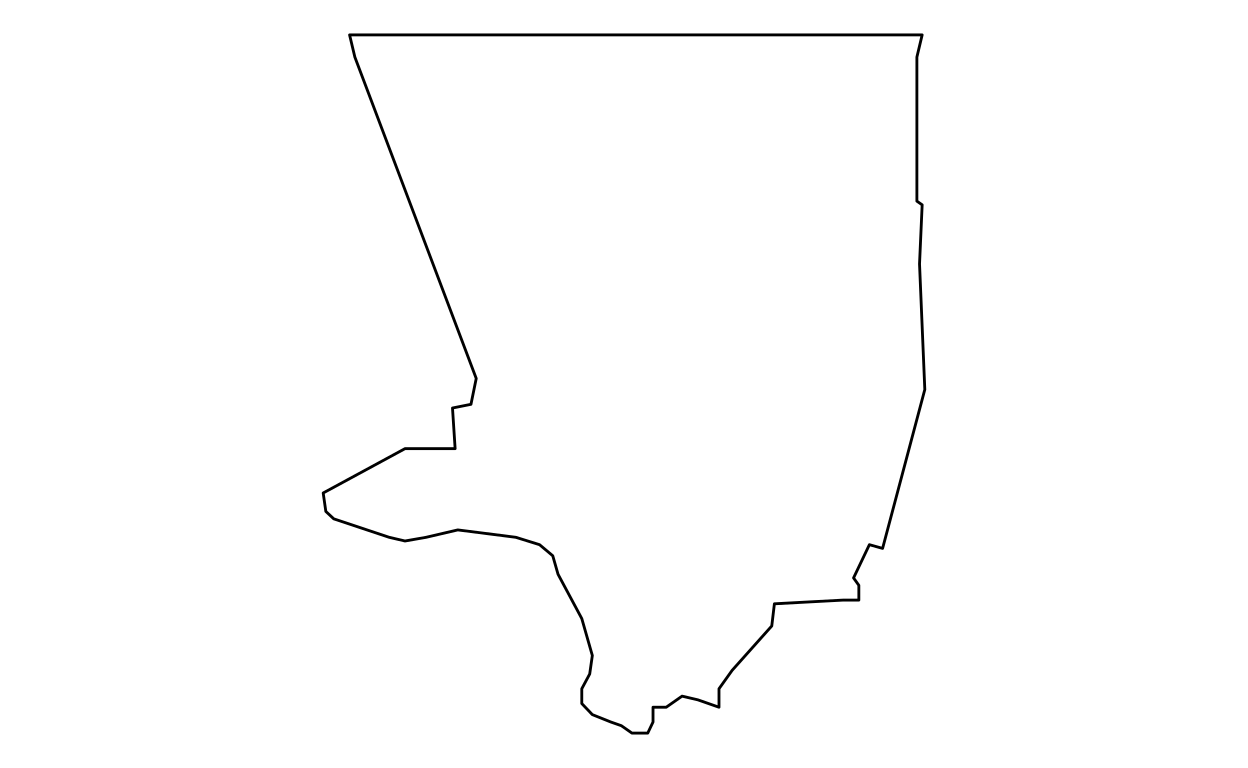### San Francisco

``````library(ggplot2)
``````

#### Step 2: Retrieve data

``````usa_counties <- map_data("county")
california_counties <- subset(usa_counties, region == "california")

sanFrancisco <- subset(california_counties, subregion == "san francisco")
``````

#### Step 3: Create maps

``````ggplot(data = sanFrancisco, aes(x = long, y = lat, group = group)) +
geom_polygon(fill = "white", color = "black") +
coord_fixed(1.4) +
theme_void()
``````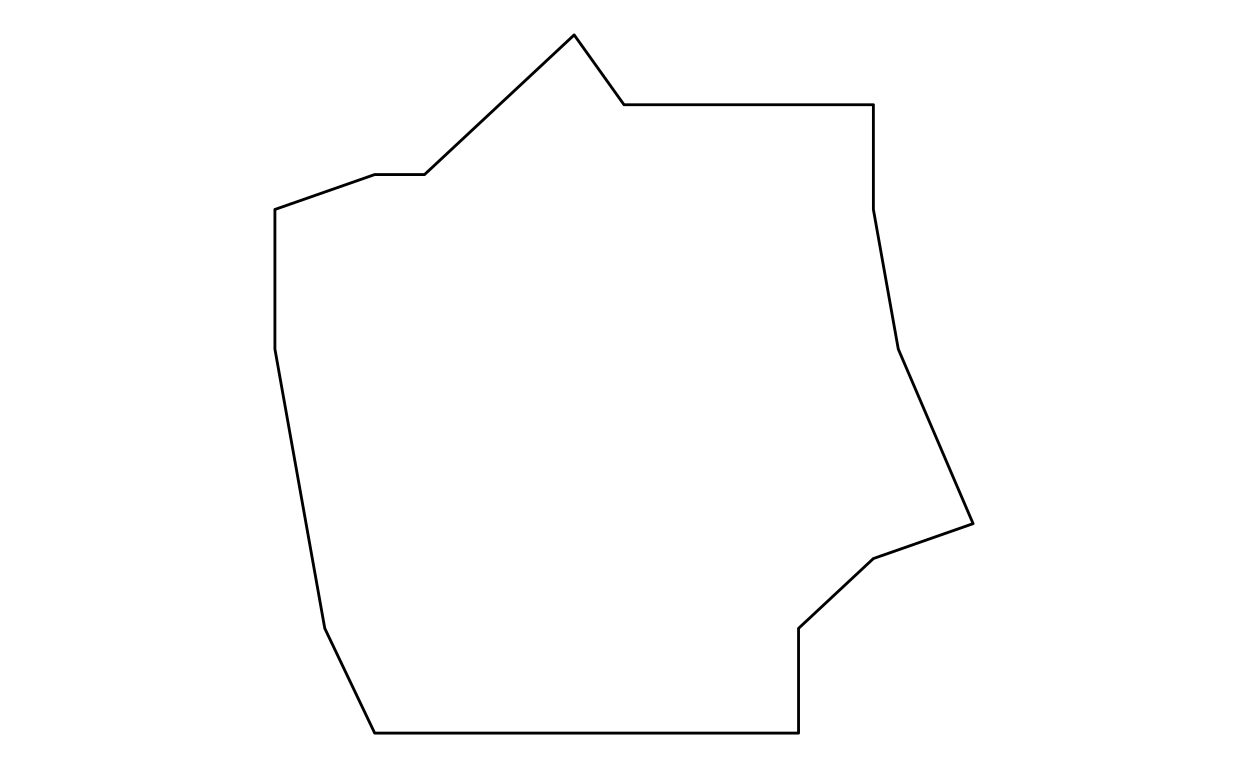## Associations and Agreements

### European Union

``````library(ggplot2)
``````

#### Step 2: Retrieve data

``````world <- map_data("world")

european.union.countries <- c("Bulgaria","Cyprus","Estonia","Finland","Greece","Ireland","Latvia",
"Lithuania","Luxembourg","Malta","Romania" ,"Sweden","Portugal",
"Spain", "France", "Switzerland", "Germany","Austria", "Belgium",
"UK", "Netherlands", "Denmark", "Poland", "Italy", "Croatia",
"Slovenia", "Hungary", "Slovakia", "Czech republic")

european.union <- map_data("world", region = european.union.countries)
``````

#### Step 3: Create maps

``````ggplot(european.union, aes(x = long, y = lat)) +
geom_polygon(aes(group = group), fill = "white", color="black") +
coord_fixed(1.2) +
theme_void()
``````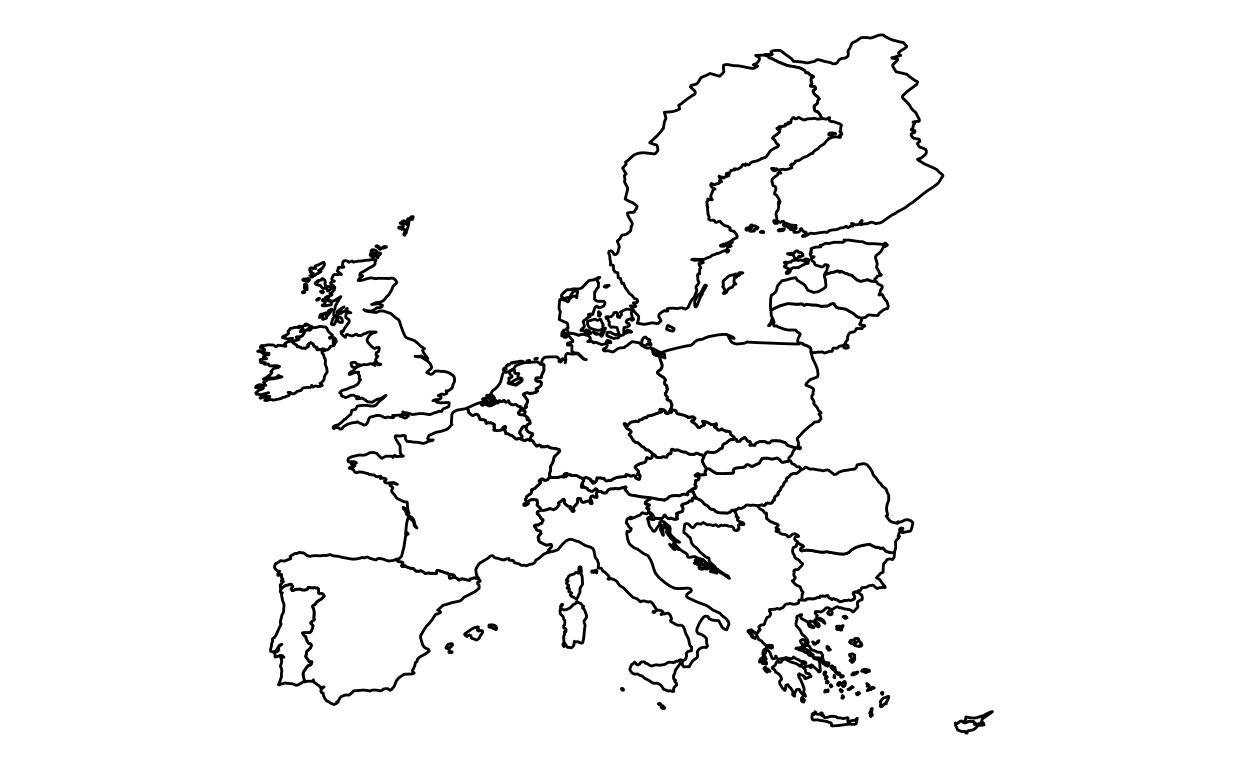### NAFTA

``````library(ggplot2)
``````

#### Step 2: Retrieve data

``````world <- map_data("world")

NAFTA <- map_data("world", region = NAFTA.countries)
``````

#### Step 3: Create maps

``````ggplot(NAFTA, aes(x = long, y = lat, group = group)) +
geom_polygon(fill = "white", color="black") +
coord_fixed(1.2, xlim = c(-175,-55)) +
theme_void()
``````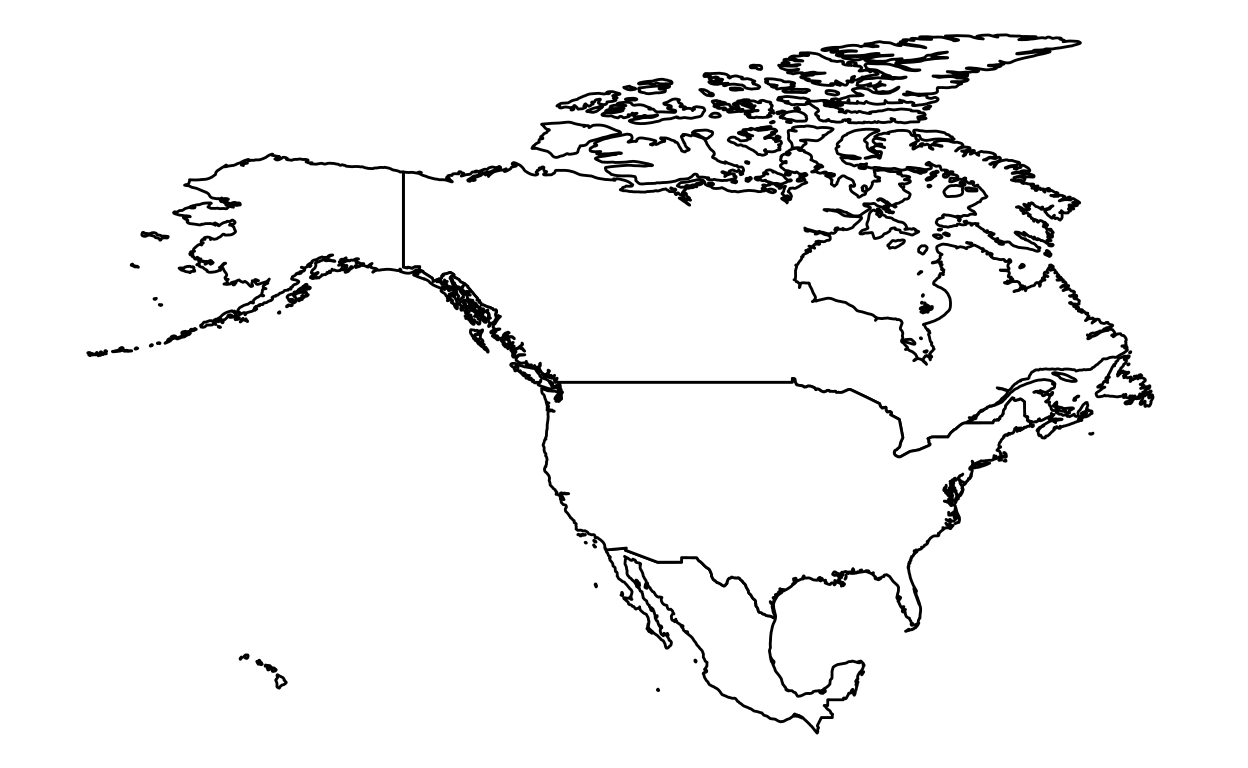## Campuses

### SKEMA

``````library(ggplot2)
``````

#### Step 2: Retrieve data

``````world <- map_data("world")

world <- subset(world, region != "Antarctica")
world <- subset(world, region != "French Southern and Antarctic Lands")

skema.campuses <- subset(world, region %in% c("France", "USA", "China", "South Africa", "Brazil"))

skema.campuses <- subset(skema.campuses, !(subregion %in% c("Alaska")))
``````

#### Step 3: Create maps

``````# Produce a World map
ggplot(data = world, aes(x = long, y = lat, group = group)) +
geom_polygon(fill = "white", color = "black", size = 0.3) +
geom_polygon(data = skema.campuses, fill = "gray", color = "black", size = 0.3) +
coord_fixed(1.5) +
theme_void()
``````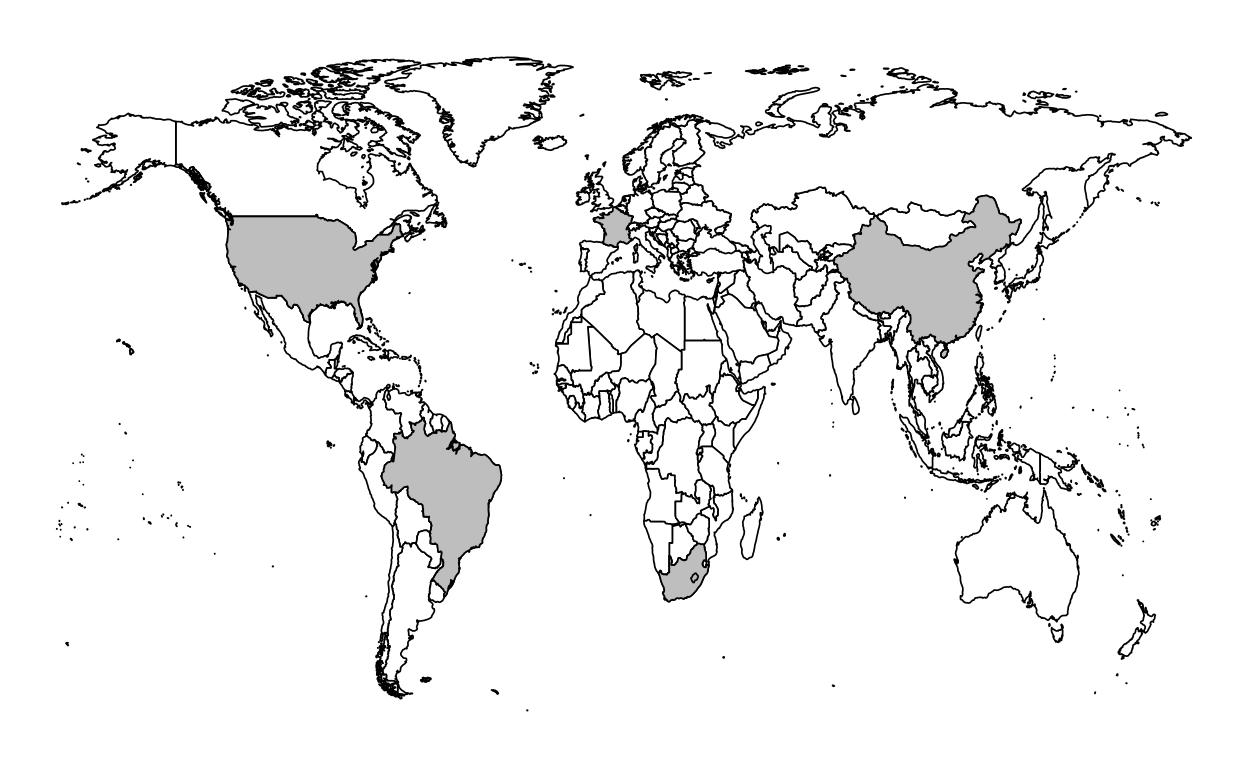### Citation

`Warin (2019, Aug. 16). www.warin.ca: [R Course] Data Visualization with R: Maps. Retrieved from https://warin.ca/posts/rcourse-datavisualizationwithr-maps/`
```@misc{warin2019[r,